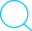# 操作系统---信号量、管程

## PV操作，管程

Posted by Kingtous on October 14, 2019

1
2
3
4
5
6
7
8
9
10
11
12
13
14
15
16
17
18
19
20
21
22
23
24
25
26
27
28
29
// operate count
// 1. lock count
// 2. if <read counter> == 0 then lock<writer> // if current reader is the first one to read the file, then it needs see if there is writers, and lock them.
// 3. unlock count

// 5. lock count
// 6. if <read counter> == 0 then unlock<writer> // if current reader is the first one to read the file, then it needs see if there is writers, and unlock them.
// 7. unlock count
}

void Writer(){
// 1. lock writer
// 2. custom write operations
// 3. wakeUp writer
}

// SYSTEM FUNCTIONS BELOW
void wait(value){
// 1. value = value - 1
// 2. if value < 0 then block value queue
}

void signal(value){
// 1. value = value + 1
// 2. if value <= 0 then wakeUp value queue
}


#### 2. (Monitors)

Monitors handle all the P-V Operations, although cut down the complicated P-V Operations in user’s applications, it may cause less efficiency.

• Only One Process in One Exact Time, use Queue to row requests.
• User’s Applications can access synchronized devices using interface provided by Monitors.
1
2
3
4
5
6
7
8
9
10
11
12
13
14
15
void put(item){
// 1. if count>=0 then cwait(notFull)
// 2. add item in queue
// 3. if notEmpty then csignal(notEmpty)
}

void producer(){
// 1. produce
// 2. put
}

void consumer(){
// 1. get
// 2. consume
}